'); }

# 2021蓝帽杯_calc

## 0x2

1. 先获得能被ida反编译且可读性较高的文件，通过ida分析大致操作，
2. 如果简单就直接推出flag，反之通过Chrome调试去分析，通过设置断点，多次调试确定判断位置，动调分析。

wasm2c下载后，通过wasm2c将wasm转换成.c文件和.h文件（进入到calc目录

``````[wasm2c目录] [文件名.wasm] -o [转换后的文件名.c]
``````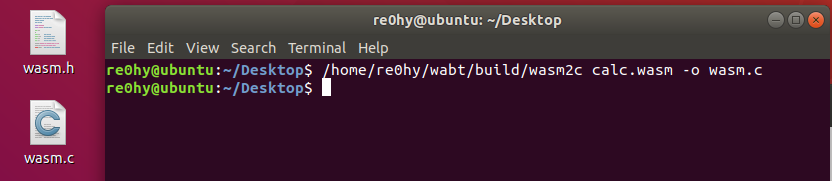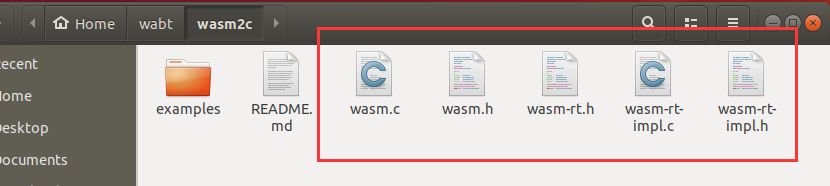``````gcc -c wasm.c -o calc.o
``````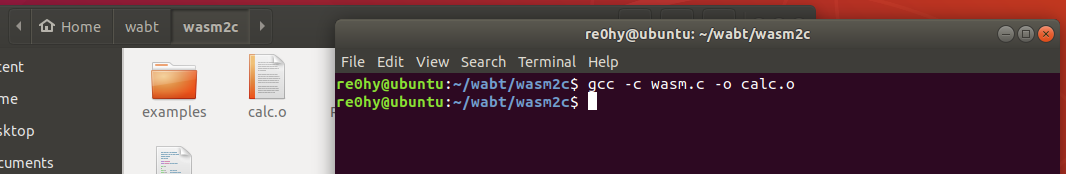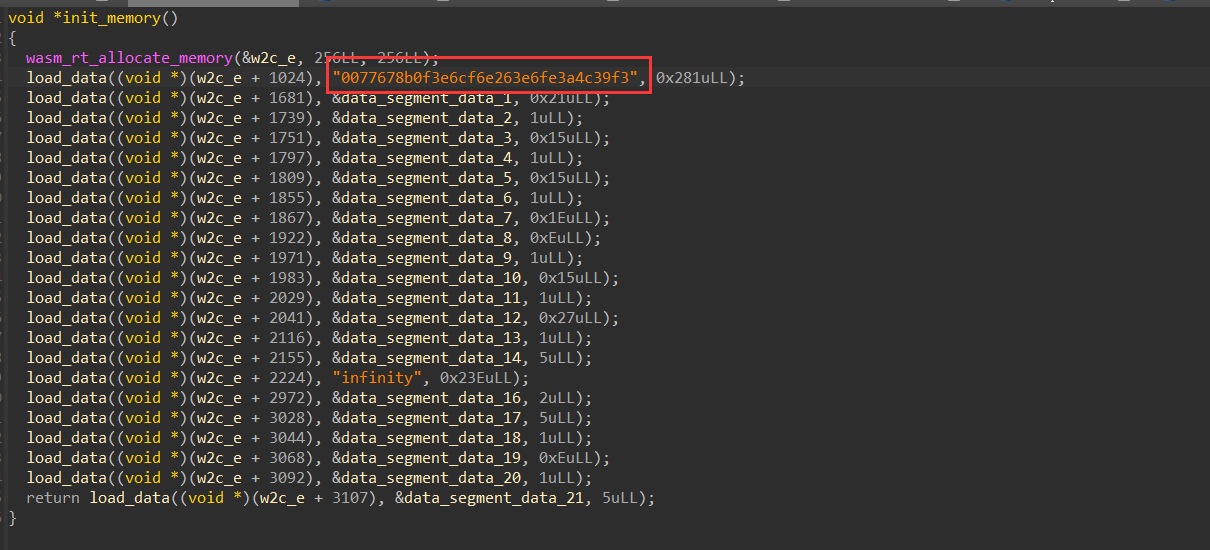``````__int64 (__fastcall *init_exports())()
{
__int64 (__fastcall *result)(); // rax

Z_e = (__int64)&w2c_e;
Z_fZ_vv = (__int64)w2c_f;
Z_gZ_iii = (__int64)w2c_g;
Z_hZ_ii = (__int64)w2c_h;
Z_i = (__int64)&w2c_i;
Z_jZ_iv = (__int64)w2c_j;
Z_kZ_vi = (__int64)w2c_k;
result = w2c_l;
Z_lZ_ii = (__int64)w2c_l;
return result;
}
``````

``````_BOOL8 __fastcall w2c_h(unsigned int a1)
{
unsigned int v1; // eax
int v3; // [rsp+Ch] [rbp-14h]
unsigned int v4; // [rsp+10h] [rbp-10h]

if ( ++wasm_rt_call_stack_depth > 0x1F4u )
wasm_rt_trap(7LL);
w2c_g0 -= 128;
v4 = w2c_g0;
w2c_f81(a1, (unsigned int)w2c_g0);
v3 = w2c_f45(v4);
if ( v3 )
v1 = 0x43D;
else
v1 = 0x435;
w2c_f29(v1);
w2c_g0 = v4 + 128;
--wasm_rt_call_stack_depth;
return v3 == 0;
}
``````

0x400多的数一般是字符串的偏移量（忘记在哪里看到的知识点了，可能有错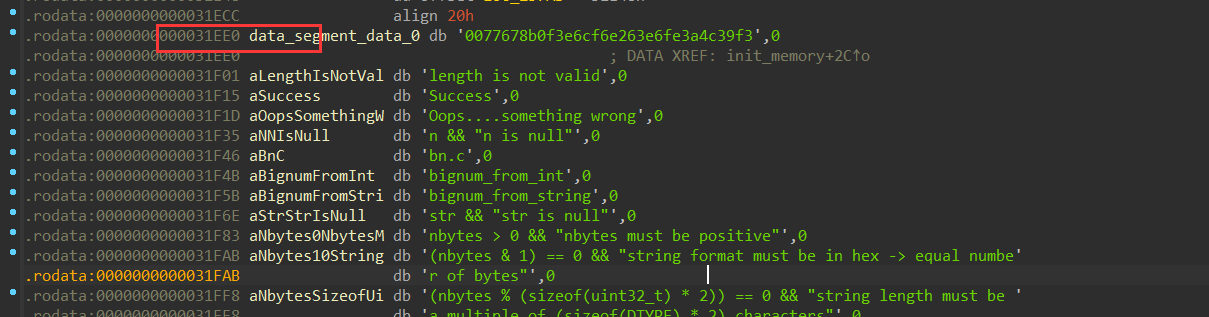``````0x31EE0-0x400+0x43D=0x31F1D
``````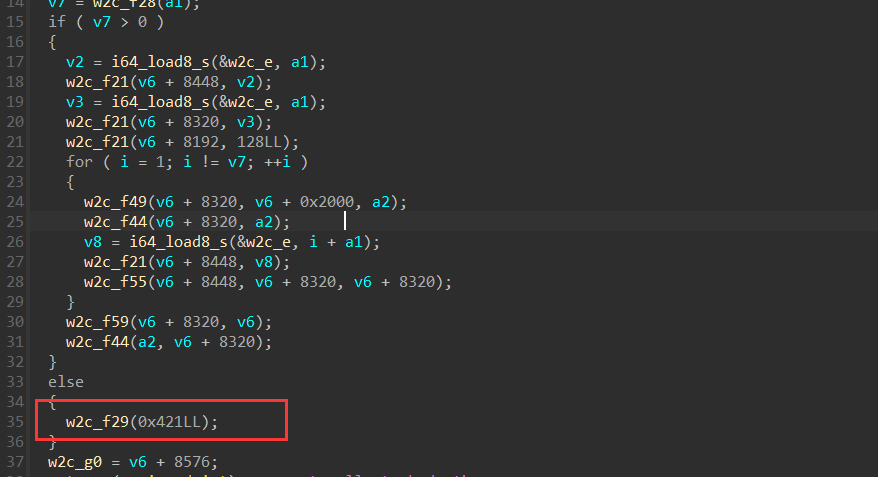``````python -m http.server -b localhost
``````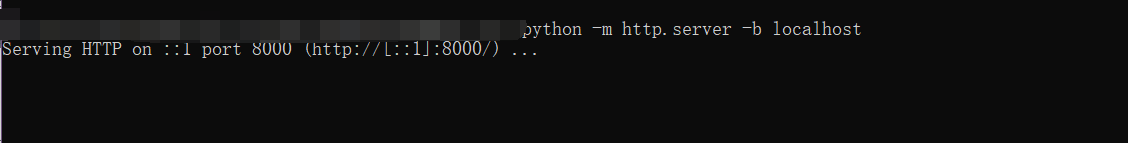``````http://localhost:8000/calc.html
``````

F12，选择Sources，在calc.html判断位置下断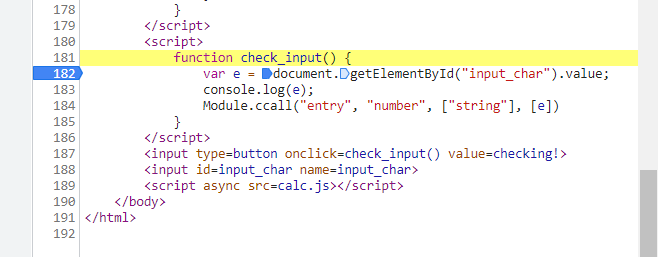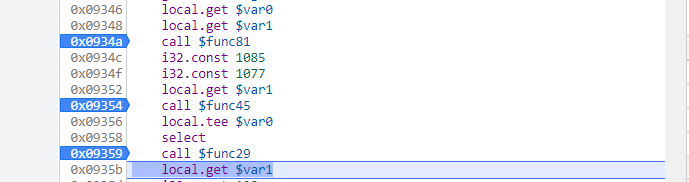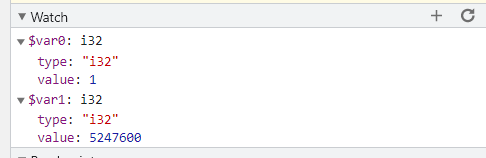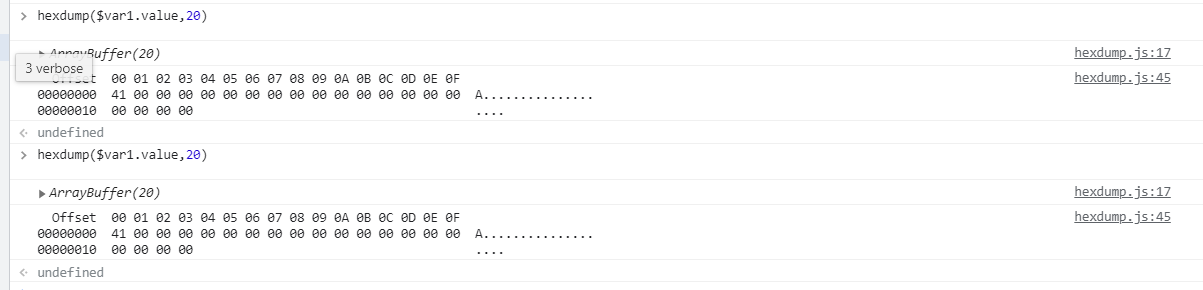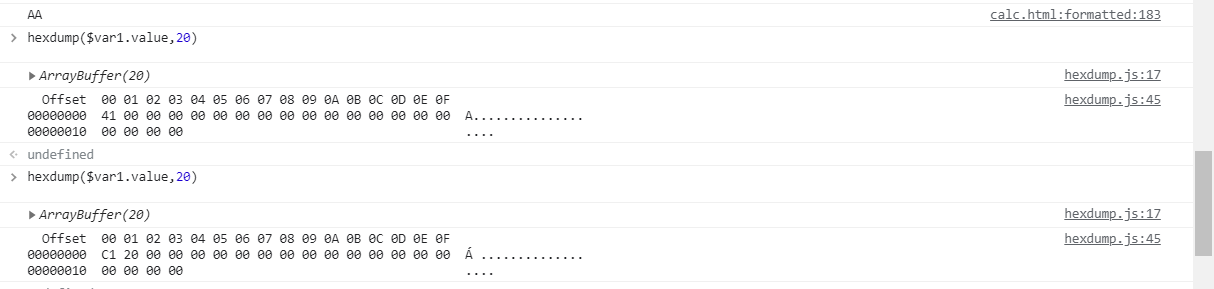``````flag | flag<<7 | flag<<14 ....
``````

``````cmp_data = [0xF3, 0x39, 0x4C, 0x3A, 0xFE, 0xE6, 0x63, 0xE2, 0xF6, 0x6C, 0x3E, 0x0F, 0x8B, 0x67, 0x77][::-1]
s = ''.join(bin(a)[2:].zfill(8) for a in cmp_data)[1:]
for i in range(0, len(s), 7):
print(chr(int(s[i:i+7], 2)), end='')
``````

``````flag{w3bass3mb1y_cR0ss}
``````

目录